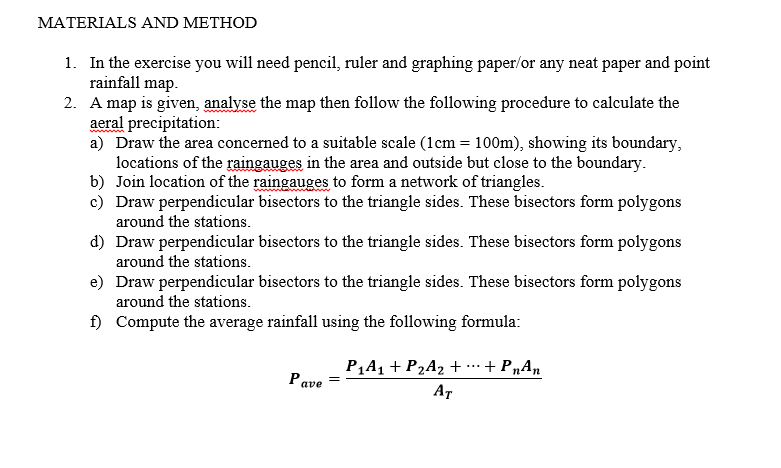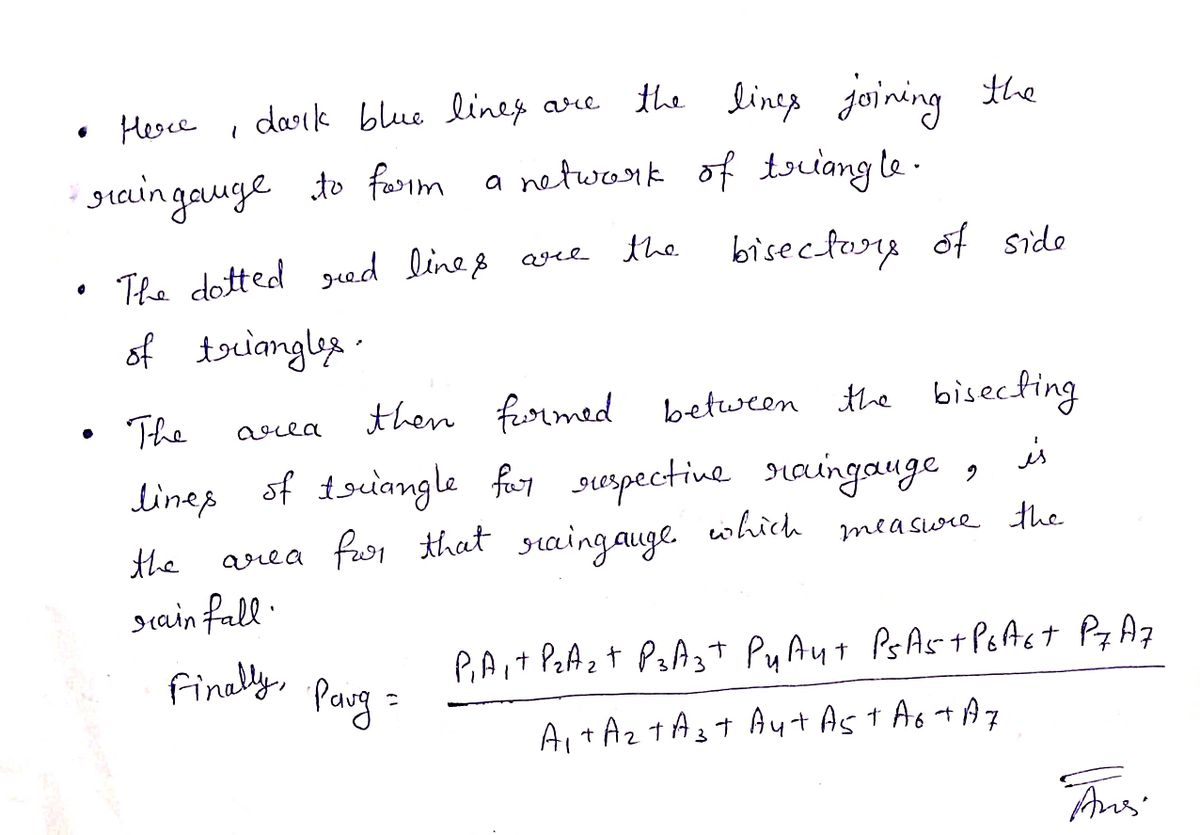# MATERIALS AND METHOD 1. In the exercise you will need pencil, ruler and graphing paper/or any neat paper and point rainfall map. 2. A map is given, analyse the map then follow the following procedure to calculate the aeral precipitation: a) Draw the area concerned to a suitable scale (1cm= 100m), showing its boundary, locations of the raingauges in the area and outside but close to the boundary. b) Join location of the raingauges to form a network of triangles. c) Draw perpendicular bisectors to the triangle sides. These bisectors form polygons around the stations. d) Draw perpendicular bisectors to the triangle sides. These bisectors form polygons around the stations. e) Draw perpendicular bisectors to the triangle sides. These bisectors form polygons around the stations. f) Compute the average rainfall using the following formula:

Question-AnswerCategory: Civil EngineeringMATERIALS AND METHOD 1. In the exercise you will need pencil, ruler and graphing paper/or any neat paper and point rainfall map. 2. A map is given, analyse the map then follow the following procedure to calculate the aeral precipitation: a) Draw the area concerned to a suitable scale (1cm= 100m), showing its boundary, locations of the raingauges in the area and outside but close to the boundary. b) Join location of the raingauges to form a network of triangles. c) Draw perpendicular bisectors to the triangle sides. These bisectors form polygons around the stations. d) Draw perpendicular bisectors to the triangle sides. These bisectors form polygons around the stations. e) Draw perpendicular bisectors to the triangle sides. These bisectors form polygons around the stations. f) Compute the average rainfall using the following formula:

### MATERIALS AND METHOD 1. In the exercise you will need pencil, ruler and graphing paper/or any neat paper and point rainfall map. 2. A map is given, analyse the map then follow the following procedure to calculate the aeral precipitation: a) Draw the area concerned to a suitable scale (1cm= 100m), showing its boundary, locations of the raingauges in the area and outside but close to the boundary. b) Join location of the raingauges to form a network of triangles. c) Draw perpendicular bisectors to the triangle sides. These bisectors form polygons around the stations. d) Draw perpendicular bisectors to the triangle sides. These bisectors form polygons around the stations. e) Draw perpendicular bisectors to the triangle sides. These bisectors form polygons around the stations. f) Compute the average rainfall using the following formula: